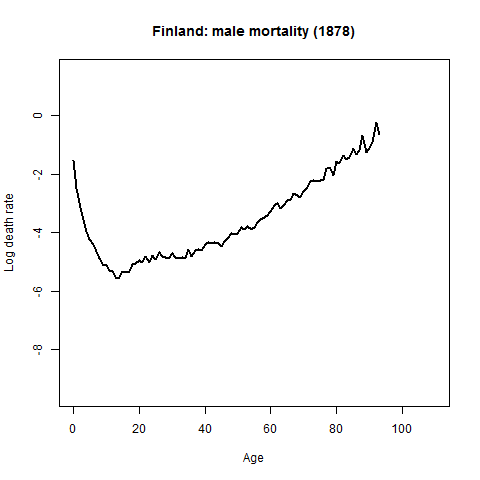# Mortality, fertility and migration

What is the most informative way to present statistical dataset or actually time series data? Most convient way is to animate this data but how to do that with fastest and easiest way? Now I want to present one way to do that. Rob J Hyndman presented this data and animation technology in his blog on October 2010. Professor Hyndman presented French mortality data and how to utilize demography R-library.In this example I will introduce how to present mortality, fertility and migration data from Finland. As data source I will use data from here: http://www.mortality.org/

To get data you have to register as a user in The Human Mortality Database (HMD).

Data source:
Human Mortality Database.  University of California, Berkeley (USA), and Max Planck Institute for Demographic Research (Germany). Available at www.mortality.org or www.humanmortality.de (data downloaded on 17.07.2012).

Finnish death rate (mortality 1x1) data table name is Mx_1x1.txt
and address is http://www.mortality.org/hmd/FIN/STATS/Mx_1x1.txt

Of course you could also use hmd function to get data from HMD (included in  demographic library) ie.

norway <- hmd.mx("NOR", "user", "pass", "Norway")
See more informaton about this from there.

Any way I downloaded Finnish data (23 Mb) as bulk transfer after registering above mentioned MHD service. In this case I do the following things:

STEP 1:

fin_mortality <- read.demogdata("data/HMD/FIN/STATS/Mx_1x1.txt",
"/data/HMD/FIN/STATS/Exposures_1x1.txt", type="mortality",
label="Finland")

STEP 2:

#NEXT NOW WE WILL DO SEVERAL PDF FILES…… 🙂

#MALE
nyears <- length(fin_mortality$year) for(i in 1:nyears) { pdf(paste("fimale",i,".pdf",sep=""),height=4,width=6.5) x <- fin_mortality if(i<nyears) x$
rate$male[,(i+1):nyears] <- NA plot(x,series="male",ylim=c(-9.5,1.5), main=paste("Finland: male mortality (",fin_mortality$year-1+i,")",
sep=""))
if(i>1)
x$rate$male[,1:(i-1)] <- NA
lines(x,series='male',lwd=2,col=1)     dev.off() }

#NEXT NOW WE WILL DO SEVERAL PDF FILES….AGAIN. 🙂

#FEMALE

nyears <- length(fin_mortality$year) for(i in 1:nyears) { pdf(paste("fifemale",i,".pdf",sep=""),height=4,width=6.5) x <- fin_mortality if(i<nyears) x$rate$female[,(i+1):nyears] <- NA plot(x,series="female",ylim=c(-9.5,1.5), main=paste("Finland: female mortality (",fin_mortality$year-1+i,")",
sep=""))     if(i>1)         x$rate$female[,1:(i-1)] <- NA
lines(x,series='female',lwd=2,col=1)     dev.off() }

#OK NOW LETS DO SOW TRICKS WITH LATEX

STEP 3
Note! run this latex file at the same working directory as previous R step.
In this example I use TexMakerX -program’s quick build –> and after that you will find animated pdf file from your working director.

%Save this in the same working dir Note: replace fifemale text with fimale to
produce male mortality animation.
\documentclass{article}
\usepackage{animate}
\usepackage{graphicx}
\begin{document}
\begin{center}
\centerline{\animategraphics[controls,buttonsize=0.3cm,width=12.5cm]
{6}{"fifemale"}{1}{132}}
\end{center}
\end{document}

STEP 4
Now You will find mortality pdf file animation male death rate and female death rate. Pdf file include “play” button, just press it and You will see mortality animation between year 1878 – 2009.

How to make sense with line colours? Please read this article from Hyndman and Shang. http://robjhyndman.com/papers/Rainbow5.pdf “… the colors of the curves follow the order of a rainbow, with the oldest data in red and the most recent data in violet….”

STEP 5
For Web publishing it is better to do gif animation: R and ImageMagick Note: install also these package if you encounter problems Microsoft Visual C++ 2010 Redistributable Package (x86) and Microsoft Visual C++ 2010 Redistributable Package (x64) package But before do that try to do following:   Note for some reason (I do not know why) I have to set my path variables like this: C:\Program Files\ImageMagick; So, I create ImageMagick -folder and copy convert.exe from folder C:\Program Files (x86)\ImageMagick-6.7.8-Q16 …and everything go fine now.

#In working director create a new folder and set the working directory to the new folder.

dir.create(“examples”)
setwd(“examples”)
library(animation)
library(demography)
#let’s check how many years we have nyears length(fin_mortality$year) #now we will do as many frames as years we have #MALE makeplot <- function(){ for(i in 1:nyears) { x <- fin_mortality if(i<nyears) x$rate$male[,(i+1):nyears] <- NA plot(x,series="male",ylim=c(-9.5,1.5), main=paste("Finland: male mortality (",fin_mortality$year-1+i,")",sep=""))     if(i>1)         x$rate$male[,1:(i-1)] <- NA lines(x,series='male',lwd=2,col=1) } } oopt = ani.options(interval = 0, nmax = nyears) saveMovie(makeplot(),interval = 0.1, width = 580, height = 400) ani.options(oopt) 

#FEMALE makeplot <- function(){ for(i in 1:nyears) {     x <- fin_mortality     if(i<nyears)         x$rate$female[,(i+1):nyears] <- NA     plot(x,series="female",ylim=c(-9.5,1.5),         main=paste("Finland: female mortality (",fin_mortality$year-1+i,")",sep="")) if(i>1) x$rate\$female[,1:(i-1)] <- NA     lines(x,series='female',lwd=2,col=1) } } oopt = ani.options(interval = 0, nmax = nyears) saveMovie(makeplot(),interval = 0.1, width = 580, height = 400) ani.options(oopt)